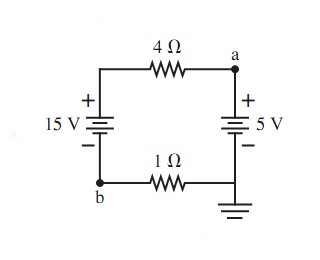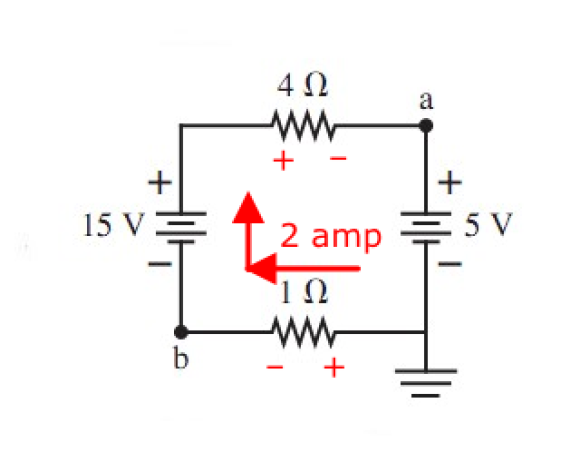# In the following figure, what is the value of the potential at points a and b? (Figure 1) Will give best answer?

+1 vote

In the following figure, what is the value of the potential at points a and b?*Please explain each step and why each step takes place. Thanks in advance.

+1 vote
by
edited by

update: Assuming you want voltage with respect to ground.

point a is +5V

Current in loop is CW and is equal to (15–5)v / (4+1)Ω = 10/5 = 2 amps

voltage drop across 1Ω resistor is E = IR = 2 volts

so Vb = –2v

edit: to elaborate a bit on that last number:

start at ground and go CW around the loop. Voltage at point a is –2 volts

next point is –2+15 = +13.

next point is –2+12–8 = +5v

next point is back to ground, 0v.Lorem ipsum dolor sit amet, consectetur adipiscing elit, sed do eiusmod tempor incididunt ut labore et dolore magna aliqua. Ut enim ad minim veniam, quis nostrud exercitation ullamco laboris nisi ut aliquip ex ea commodo consequat. Duis aute irure dolor in reprehenderit in voluptate velit esse cillum dolore eu fugiat.